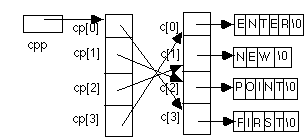1. ```a  = ( (b  *  c)  ==  2 )
a  = ( ( b  &&  ( a  >  z ) ) ?  ( x  =  y ) :  z )
a  = ( ( s  .  f ) + ( x  .  y ) )
a  = ( b  >> ( 2  +  4 ) )
c  = ( getchar()  ==  EOF )
```
2. It does not produce a segmentation fault because the "and" operator, &&, evaluates only as many operands as needed to determine the value of the expression. This means that, in the above, if p is NULL, the left operand of && is false, and so the value of the entire && expression is false, regardless of the value of the right-hand operator. So the right-hand operator is never evaluated, meaning the value of *p is never accessed, meaning the pointer p is not dereferenced. As this is what caused the segmentation fault in the original program, no such segmentation fault occurs in the second.

3. In all these answers, remember that control flows from the relevant case either to the end of the switch statement or until a break statement is encountered. So:
1. ```baa
meep
sproing
```
2. ```meep
sproing
```
3. As there is no case for 0, there is no output; the switch statement is skipped.

4. ```/* Count the number of a's  */
/* in a line given as input */

#include <stdio.h>
#include <ctype.h>

void main(void)
{
int num_a = 0;
int c;

while((c = getchar()) != '\n') {
while(isdigit(c))
;
if (c == 'a')
num_a++;
}
printf("%d\n", num_a);
exit(0);
}
```

Incidentally, there is a bug in this program if c is a digit; the program enters an infinite loop. Note that in my solution I have preserved the bug (the question didn't say to debug the program ...)

5. Let's first take the hint and draw a picture:The first printf increments cpp and dereferences it twice; so, after the ++, the variable cpp is cp+1, and *cpp is c + 2 or &c, giving **cpp as c, or "POINT". The picture now looks like:The second printf 's second argument is (*(--(*(++cpp))))+3; so, cpp now becomes cp+2, *++cpp is c+1 or &c, --(*(++cpp)) stores (c+1)-1 or c in cp, and (*(--(*(++cpp)))) is c. Hence, (*(--(*(++cpp))))+3 is &c+3, or "ER". Again, the picture has changed slightly:In the third printf, *cpp[-2]+3 is (*(cpp[-2]))+3, or (*(*(cpp-2)))+3; the value stored in the variable cpp is cp+2, so *(cpp-2) is *(cp+2-2) or *cp, or c+3 or &c, or "FIRST"; hence (*(*(cpp-2)))+3 is &c+3 or "ST".

In the fourth printf, cpp[-1][-1] is (*(*cpp-1)-1), or (*(cp+2-1)-1), or (*(cp+1)-1), or (c+2-1), or c+1, or &c; so, cpp[-1][-1]+1 is &c+1, or "EW".

Putting this into the formats, the code fragment prints:

```POINTER STEW
```
followed by a newline.

6. variablevalue if min is macrovalue if min is function
a43
b33
c55
d43
e33
Evaluating the functions is straightforward: the incrementing occurs once. For the macro, though, we need to look at the full expansion.

For min a macro, the fourth line becomes:

```d = ((++a) > b) ? (b) : (++a)
```
Now, as a is 2 and b is 3, the conditional is false (as 3 == 3), so ++a is evaluated, setting a to 4 and returning 4 as the expression value. For the fifth line, we have
```e = ((c++) > b) ? (b) : (c++)
```
giving a true conditional (as 4 > 3; note c is incremented after its value is used), whence the value of b, or 3, is assigned to e.

7. variable final value
a 2
arr 3
arr 5
arr 5
b &arr
c &arr
d 2
e 3
f 4

All parameters are passed by value, not reference.

As testandinc treats its parameter as an integer, and not an integer pointer, a's value cannot change. As testandinc returns the value of its parameter (because the ++ follows the x, x is incremented after its value is used), it also returns the value of its parameter. The ++ is effectively a do-nothing operator.

The second function, p1testandinc, takes a pointer as its parameter:As you can see from the picture, any changes to the parameter's value will not affect the pointer b; only the local copy of that value, stored in x. The expression *x++ is the same as *(x++); this value is 3 (as the ++ is postincrement, so it is done after x's value is used). Hence p1testandinc returns 3, and b remains arr (or &arr).

The third function is a bit different. The return value is evaluated differently; the pointer is dereferenced first, then the value x points to is incremented. (Note the parentheses change the meaning from the second function's return value.) So, not, the value of the argument on return is unchanged; but the value stored in arr, to which the argument points, is incremented from 4 to 5.

1. Use pipes:
```sed 's/:.*//' /etc/passwd | sort | uniq -u > usernames
```
2. mkdir heidi-hw1
```ls *rc .*rc